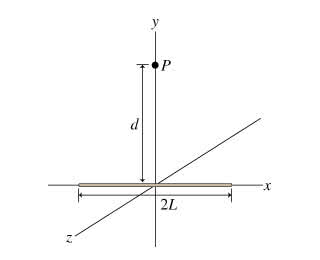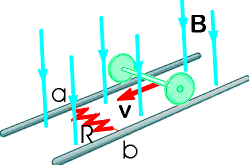Ask question

# A slab of insulating material of uniform thickness d, lying between \frac{-d}{2} to \frac{d}{2} along the x axis, extends infinitely in the y and z directions, as shown in the figure. The slab has a uniform charge density \rho. The electric field is zero in the middle of the slab, at x=0. Which of the following statements is true of the electric field E_{vec} at the surface of one side of the slab?# A slab of insulating material of uniform thickness d, lying between \frac{-d}{2} to \frac{d}{2} along the x axis, extends infinitely in the y and z directions, as shown in the figure. The slab has a uniform charge density \rho. The electric field is zero in the middle of the slab, at x=0. Which of the following statements is true of the electric field E_{vec} at the surface of one side of the slab?

Question
Otherasked 2021-04-13
A slab of insulating material of uniform thickness d, lying between $$\displaystyle{\frac{{-{d}}}{{{2}}}}$$ to $$\displaystyle{\frac{{{d}}}{{{2}}}}$$ along the x axis, extends infinitely in the y and z directions, as shown in the figure. The slab has a uniform charge density $$\displaystyle\rho$$. The electric field is zero in the middle of the slab, at x=0. Which of the following statements is true of the electric field $$\displaystyle{E}_{{{\vec}}}$$ at the surface of one side of the slab?

## Answers (1)2021-04-15
(a) Both the magnitude and the direction of Electric Field (E) are constant across the entire surface.
(b) $$\displaystyle\theta={1.57}\ {r}{a}{d}$$
(c) As implied by the fact that E(out) is not given as a function of x, this magnitude is constant everywhere outside the slab, not just at the surface.
$$\displaystyle{E}_{{{o}{u}{t}}}={\frac{{{d}\rho}}{{{2}\cdot\epsilon_{{0}}}}}$$
(d) $$\displaystyle{E}_{{\in}}={p}{h}{o}\cdot{\frac{{{X}}}{{\epsilon_{{0}}}}}$$

### Relevant Questionsasked 2021-03-30
A long, straight, copper wire with a circular cross-sectional area of $$\displaystyle{2.1}{m}{m}^{{2}}$$ carries a current of 16 A. The resistivity of the material is $$\displaystyle{2.0}\times{10}^{{-{8}}}$$ Om.
a) What is the uniform electric field in the material?
b) If the current is changing at the rate of 4000 A/s, at whatrate is the electric field in the material changing?
c) What is the displacement current density in the material in part (b)?
d) If the current is changing as in part (b), what is the magnitude of the magnetic field 6.0cm from the center of the wire? Note that both the conduction current and the displacement currentshould be included in the calculation of B. Is the contribution from the displacement current significant?asked 2021-05-20
Assume that a ball of charged particles has a uniformly distributednegative charge density except for a narrow radial tunnel throughits center, from the surface on one side to the surface on the opposite side. Also assume that we can position a proton any where along the tunnel or outside the ball. Let $$\displaystyle{F}_{{R}}$$ be the magnitude of the electrostatic force on the proton when it islocated at the ball's surface, at radius R. As a multiple ofR, how far from the surface is there a point where the forcemagnitude is 0.44FR if we move the proton(a) away from the ball and (b) into the tunnel?asked 2021-04-27
A charged wire of negligible thickness has length 2L units and has a linear charge density ?. Consider the electric field E? at the point P, a distance d above the midpoint of the wire.asked 2021-04-22
Nerve cells are long, thin cylinders along which electric disturbances (nerve impulses) travel. The cell membrane if a typical nerve cell consists of an inner and outer wall separated by a distance of $$\displaystyle{0.10}\mu{m}$$. The electric field within the cell membrane is $$\displaystyle{7.0}\cdot{10}^{{{5}}}$$ N/C. Approximating the cell membrane as a parallel plate capacitor, determine the magnitude of the charge density on the inner and outer cell walls.asked 2021-02-08
An infinitely long rod of radius R carries a uniform volume charge density PKS\rho\ (\rho > 0)ZSK.
a) Show how to use Gauss' Law to prove that the electric field inside this rod points radially outward and has magnitude.
$$\displaystyle{E}={\frac{{\rho\cdot{r}}}{{{2}\cdot\epsilon_{{0}}}}}$$
b) Integrate the electric field over an appropriate displacement to find the potential difference from the rod's surface to its axis. State explicity which of those two locations is at the higher potential.asked 2021-05-18
The student engineer of a campus radio station wishes to verify the effectivencess of the lightning rod on the antenna mast. The unknown resistance $$\displaystyle{R}_{{x}}$$ is between points C and E. Point E is a "true ground", but is inaccessible for direct measurement because the stratum in which it is located is several meters below Earth's surface. Two identical rods are driven into the ground at A and B, introducing an unknown resistance $$\displaystyle{R}_{{y}}$$. The procedure for finding the unknown resistance $$\displaystyle{R}_{{x}}$$ is as follows. Measure resistance $$\displaystyle{R}_{{1}}$$ between points A and B. Then connect A and B with a heavy conducting wire and measure resistance $$\displaystyle{R}_{{2}}$$ between points A and C.Derive a formula for $$\displaystyle{R}_{{x}}$$ in terms of the observable resistances $$\displaystyle{R}_{{1}}$$ and $$\displaystyle{R}_{{2}}$$. A satisfactory ground resistance would be $$\displaystyle{R}_{{x}}{<}{2.0}$$ Ohms. Is the grounding of the station adequate if measurments give $$\displaystyle{R}_{{1}}={13}{O}{h}{m}{s}$$ and R_2=6.0 Ohms?asked 2021-02-23
A 0.30 kg ladle sliding on a horizontal frictionless surface isattached to one end of a horizontal spring (k = 500 N/m) whoseother end is fixed. The ladle has a kinetic energy of 10 J as itpasses through its equilibrium position (the point at which thespring force is zero).
(a) At what rate is the spring doing work on the ladle as the ladlepasses through its equilibrium position?
(b) At what rate is the spring doing work on the ladle when thespring is compressed 0.10 m and the ladle is moving away from theequilibrium position?asked 2021-05-02
Suppose electrons enter the uniform electric field midwaybetween two plates, moving at an upward 45 degree angle as shown.What maximum speed can the electrons have if they are to avoidstriking the upper plate. Ignore fringing of the field. Upper plate is negatively charged bottom plate is positivelycharged length of plate is 6cm and distance from top of top plateto bottom of bottom plate is 1cm.
$$\displaystyle{E}={5.0}\times{10}^{{3}}\frac{{N}}{{C}}$$asked 2021-03-21
In the figure below, the rolling axle, 1.43 m long, is pushed along horizontal rails at a constant speed v = 3.36 m/s.A resistor R = 0.325 ohm is connected to the rails at points a and b, directly opposite each other. (The wheels make good electrical contact with the rails, and so the axle, rails, and R form a closed-loop circuit. The only significant resistance in the circuit is R.) There is a uniform magnetic field B = 0.0850 T vertically downward. Calculate the induced current I in the resistor and what horizontal force F is required to keep the axle rolling at constant speed?asked 2021-04-13
A fish is swimming in water inside a thin spherical glass bowlof uniform thickness. Assuming the radius of curvature of thebowl is 25cm, locate the image of the fish if the fish is located:(a) at the center of the bowl;
(b) 20.0 cm from the side of the bowl between the observer andthe center of the bowl.
...